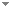Switch to: References

# Reverse mathematics, computability, and partitions of trees

Journal of Symbolic Logic 74 (1):201-215 (2009)

 Hindman’s Theorem for Sums Along the Full Binary Tree, $$\sigma ^0_2$$ Σ 2 0 -Induction and the Pigeonhole Principle for Trees. [REVIEW]Daniele Tavernelli & Lorenzo Carlucci - 2022 - Archive for Mathematical Logic 61 (5-6):827-839.We formulate a restriction of Hindman’s Finite Sums Theorem in which monochromaticity is required only for sums corresponding to rooted finite paths in the full binary tree. We show that the resulting principle is equivalent to Σ20\documentclass[12pt]{minimal} \usepackage{amsmath} \usepackage{wasysym} \usepackage{amsfonts} \usepackage{amssymb} \usepackage{amsbsy} \usepackage{mathrsfs} \usepackage{upgreek} \setlength{\oddsidemargin}{-69pt} \begin{document}$$\Sigma ^0_2$$\end{document}-induction over RCA0\documentclass[12pt]{minimal} \usepackage{amsmath} \usepackage{wasysym} \usepackage{amsfonts} \usepackage{amssymb} \usepackage{amsbsy} \usepackage{mathrsfs} \usepackage{upgreek} \setlength{\oddsidemargin}{-69pt} \begin{document}$$\mathsf {RCA}_0$$\end{document}. The proof uses the equivalence of this Hindman-type theorem with the Pigeonhole Principle for trees TT1\documentclass[12pt]{minimal} \usepackage{amsmath} \usepackage{wasysym} \usepackage{amsfonts} \usepackage{amssymb} \usepackage{amsbsy} \usepackage{mathrsfs} (...)No categories Direct download (2 more)     Export citationBookmarkHindman’s theorem for sums along the full binary tree, $$\Sigma ^0_2$$ Σ 2 0 -induction and the Pigeonhole principle for trees. [REVIEW]Lorenzo Carlucci & Daniele Tavernelli - 2022 - Archive for Mathematical Logic 61 (5):827-839.We formulate a restriction of Hindman’s Finite Sums Theorem in which monochromaticity is required only for sums corresponding to rooted finite paths in the full binary tree. We show that the resulting principle is equivalent to \-induction over \. The proof uses the equivalence of this Hindman-type theorem with the Pigeonhole Principle for trees \ with an extra condition on the solution tree. No categories Direct download (3 more)   Translate     Export citationBookmarkRamsey’s Theorem for Trees: The Polarized Tree Theorem and Notions of Stability. [REVIEW]Damir D. Dzhafarov, Jeffry L. Hirst & Tamara J. Lakins - 2010 - Archive for Mathematical Logic 49 (3):399-415.We formulate a polarized version of Ramsey’s theorem for trees. For those exponents greater than 2, both the reverse mathematics and the computability theory associated with this theorem parallel that of its linear analog. For pairs, the situation is more complex. In particular, there are many reasonable notions of stability in the tree setting, complicating the analysis of the related results. Direct download (3 more)     Export citationBookmark3 citations Partitions of Trees and $${{\sf ACA}^\prime_{0}}$$.Bernard A. Anderson & Jeffry L. Hirst - 2009 - Archive for Mathematical Logic 48 (3-4):227-230.We show that a version of Ramsey’s theorem for trees for arbitrary exponents is equivalent to the subsystem ${{\sf ACA}^\prime_{0}}$ of reverse mathematics. Direct download (3 more)     Export citationBookmarkReverse Mathematics and Ramsey Properties of Partial Orderings.Jared Corduan & Marcia Groszek - 2016 - Notre Dame Journal of Formal Logic 57 (1):1-25.A partial ordering $\mathbb{P}$ is $n$-Ramsey if, for every coloring of $n$-element chains from $\mathbb{P}$ in finitely many colors, $\mathbb{P}$ has a homogeneous subordering isomorphic to $\mathbb{P}$. In their paper on Ramsey properties of the complete binary tree, Chubb, Hirst, and McNicholl ask about Ramsey properties of other partial orderings. They also ask whether there is some Ramsey property for pairs equivalent to $\mathit{ACA}_{0}$ over $\mathit{RCA}_{0}$. A characterization theorem for finite-level partial orderings with Ramsey properties has been proven by the (...) Direct download (3 more)     Export citationBookmarkThe Strength of the Tree Theorem for Pairs in Reverse Mathematics.Ludovic Patey - 2016 - Journal of Symbolic Logic 81 (4):1481-1499. Direct download (4 more)     Export citationBookmarkPartitions of Trees And.Bernard A. Anderson & Jeffry L. Hirst - 2009 - Archive for Mathematical Logic 48 (3-4):227-230.We show that a version of Ramsey’s theorem for trees for arbitrary exponents is equivalent to the subsystem \documentclass[12pt]{minimal} \usepackage{amsmath} \usepackage{wasysym} \usepackage{amsfonts} \usepackage{amssymb} \usepackage{amsbsy} \usepackage{mathrsfs} \usepackage{upgreek} \setlength{\oddsidemargin}{-69pt} \begin{document}$${{\sf ACA}^\prime_{0}}$$\end{document} of reverse mathematics. Direct download (2 more)     Export citationBookmarkNonstandard Models in Recursion Theory and Reverse Mathematics.C. T. Chong, Wei Li & Yue Yang - 2014 - Bulletin of Symbolic Logic 20 (2):170-200.We give a survey of the study of nonstandard models in recursion theory and reverse mathematics. We discuss the key notions and techniques in effective computability in nonstandard models, and their applications to problems concerning combinatorial principles in subsystems of second order arithmetic. Particular attention is given to principles related to Ramsey’s Theorem for Pairs. Direct download (3 more)     Export citationBookmark2 citations Open Questions in Reverse Mathematics.Antonio Montalbán - 2011 - Bulletin of Symbolic Logic 17 (3):431-454.We present a list of open questions in reverse mathematics, including some relevant background information for each question. We also mention some of the areas of reverse mathematics that are starting to be developed and where interesting open question may be found. Direct download (8 more)     Export citationBookmark25 citations 2009 North American Annual Meeting of the Association for Symbolic Logic.Alasdair Urquhart - 2009 - Bulletin of Symbolic Logic 15 (4):441-464. Direct download (2 more)     Export citationBookmark